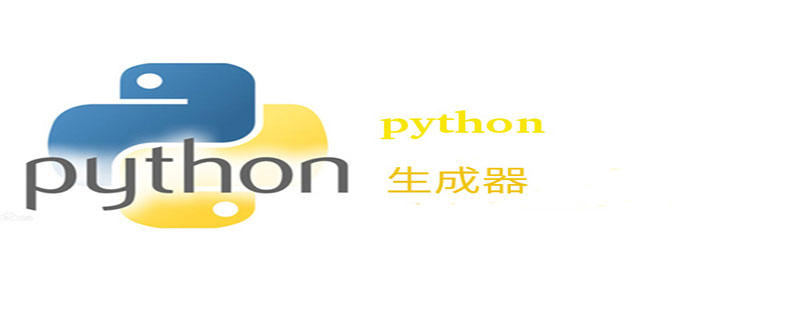Jquery中文网 www.jquerycn.cn
Jquery中文网 >  Python编程  >  Python入门  >  正文 python中的生成器到底是个啥

# python中的生成器到底是个啥

jquery中文网为您提供python中的生成器到底是个啥等资源，欢迎您收藏本站，我们将为您提供最新的python中的生成器到底是个啥资源```In : def lessthan(n):
...:     for i in range(n-1, -1, -1):
...:         yield i
...:
...:

In : for i in lessthan(5):
...:     print(i)
...:
4
3
2
1
0
In : lt = lessthan(3)

## 查看生成器对象的__iter__()和__next__()：
In : lt.__iter__?
Signature:      lt.__iter__()
Call signature: lt.__iter__(*args, **kwargs)
Type:           method-wrapper
String form:    <method-wrapper '__iter__' of generator object at 0x7fc048cb8ba0>
Docstring:      Implement iter(self).

In : lt.__next__?
Signature:      lt.__next__()
Call signature: lt.__next__(*args, **kwargs)
Type:           method-wrapper
String form:    <method-wrapper '__next__' of generator object at 0x7fc048cb8ba0>
Docstring:      Implement next(self).```

```In : lt = lessthan(3)

In : next(lt)
Out: 2

In : next(lt)
Out: 1

In : next(lt)
Out: 0

In : next(lt)
---------------------------------------------------
StopIteration      Traceback (most recent call last)
<ipython-input-37-00f31299a3f9> in <module>
----> 1 next(lt)

StopIteration:```

```In : mylist = [i*i for i in range(10)]

In : mylist
Out: [0, 1, 4, 9, 16, 25, 36, 49, 64, 81]

In : mygener = (i*i for i in range(10))

In : mygener
Out: <generator object <genexpr> at 0x7fc048be3bf8>```

```In : sum(i*i for i in range(10))
Out: 285```

sum()括号里面的i*i for i in range(10)就是一个生成器解析式，避免生成一个列表而占用过多内存。

```xvec = [10, 20, 30]
yvec = [7, 5, 3]
sum(x*y for x,y in zip(xvec, yvec))         # dot product

from math import pi, sin
sine_table = {x: sin(x*pi/180) for x in range(0, 91)}

unique_words = set(word  for line in page  for word in line.split())

valedictorian = max((student.gpa, student.name) for student in graduates)

data = 'golf'
list(data[i] for i in range(len(data)-1, -1, -1))```

Python提供了两种方式实现生成器：

（1）生成器函数

（2）生成器解析式

（3）生成器的优点

[]